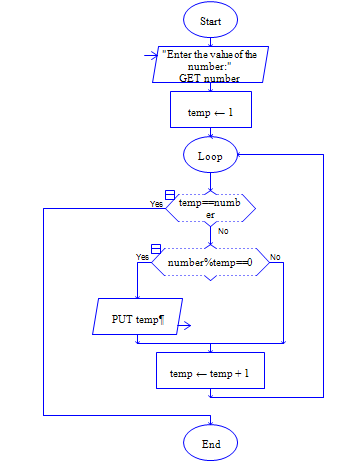# Factors of given number in Python

Write a Program to get a number n and to find the factors of n. Generally factors are the number which divides the number n.

Sample Input:

24

Sample Output:

1

2

3

4

6

12

24

#### Flow Chart DesignStrongly recommended to Solve it on your own, Don't directly go to the solution given below.

#### Program or Solution

``` #Program to Find Factors of Given number nn = int(input("Enter a Number:")) #get input n#checks which are the numbers from 1 to n/2 divides n.#No other after n/2 divides n except nfor i in range(1,n//2+1):    if n % i == 0:        print(i)#print n because every number is divisible by the same numberprint(n) ```

#### Program Explanation

input() gets the value of n from users as string, and int() coverts the same to integer.

The following for loop iterates from i = 1 to n/2, in each iteration it checks whether the n is divisible by i.

if i divides n then print i. No other number after n/2 divides n except n.

Print n at last because every number is divisible by the same number